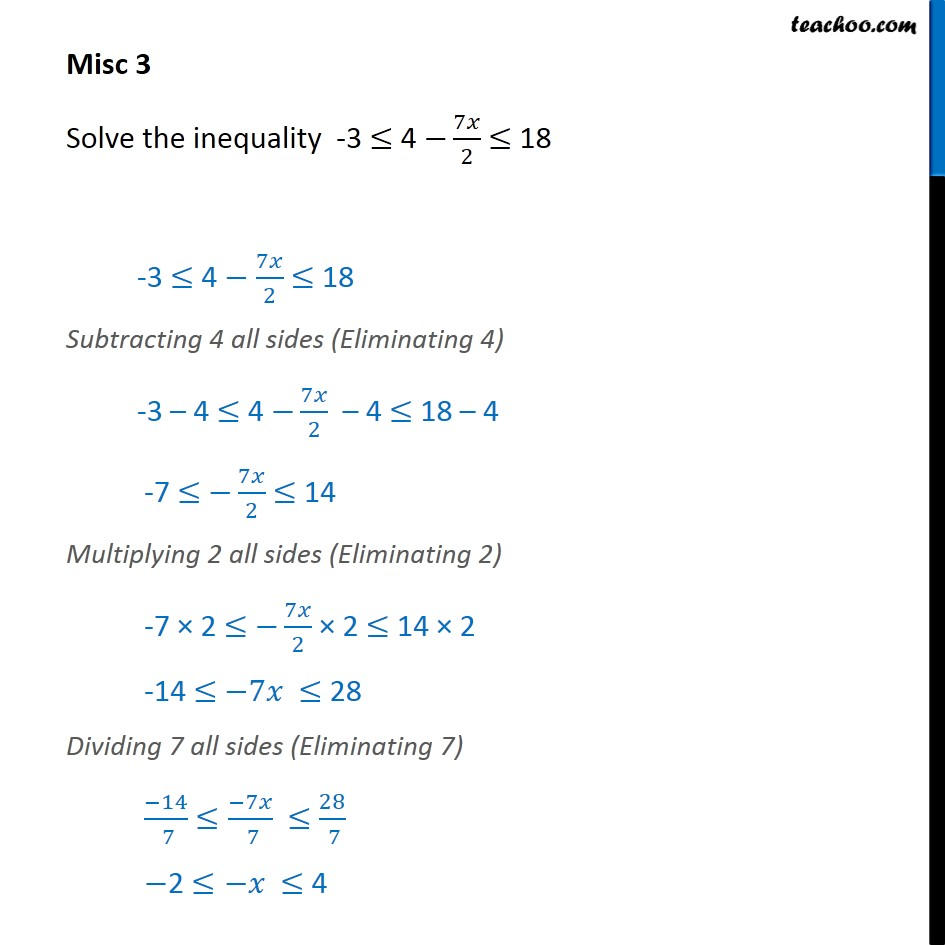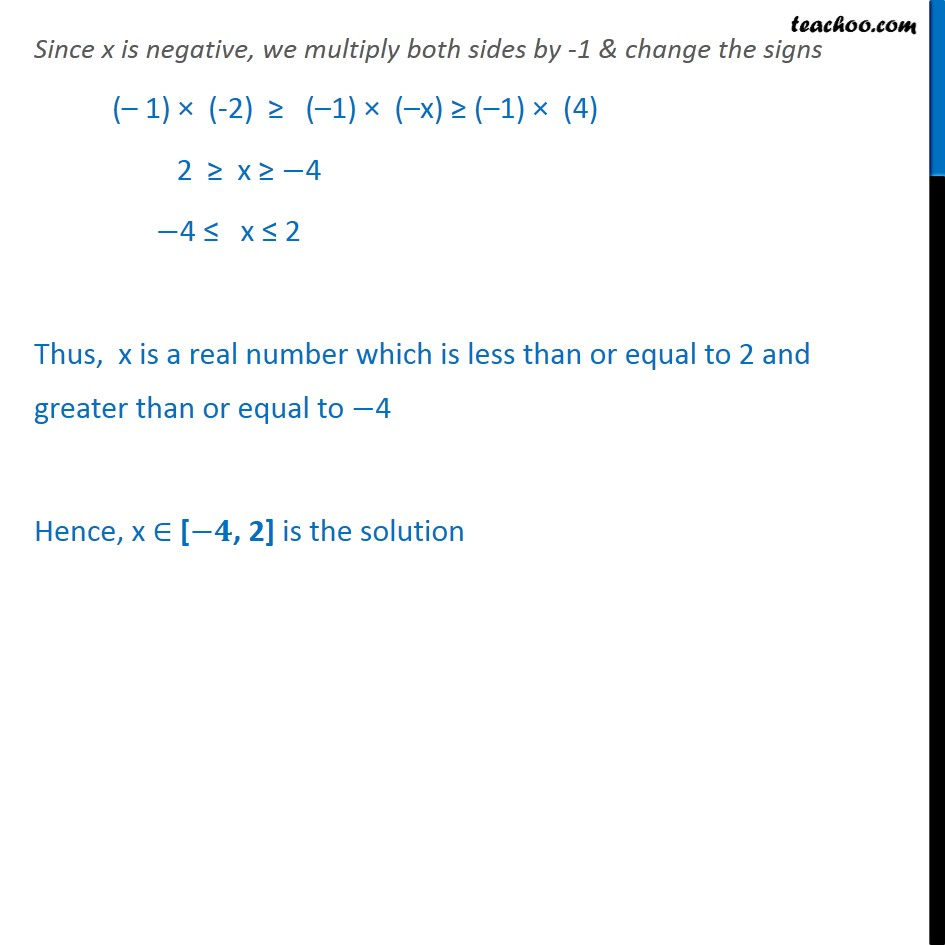Miscellaneous

Chapter 5 Class 11 Linear Inequalities
Serial order wiseLearn in your speed, with individual attention - Teachoo Maths 1-on-1 Class

### Transcript

Misc 3 Solve the inequality -3 ≤ 4 − 7𝑥﷮2﷯ ≤ 18 -3 ≤ 4 − 7𝑥﷮2﷯ ≤ 18 Subtracting 4 all sides (Eliminating 4) -3 – 4 ≤ 4 − 7𝑥﷮2﷯ – 4 ≤ 18 – 4 -7 ≤ − 7𝑥﷮2﷯ ≤ 14 Multiplying 2 all sides (Eliminating 2) -7 × 2 ≤ − 7𝑥﷮2﷯ × 2 ≤ 14 × 2 -14 ≤ −7𝑥 ≤ 28 Dividing 7 all sides (Eliminating 7) −14﷮ 7﷯ ≤ −7𝑥﷮ 7﷯ ≤ 28﷮ 7﷯ −2 ≤−𝑥 ≤ 4 Since x is negative, we multiply both sides by -1 & change the signs (– 1) × (-2) ≥ (–1) × (–x) ≥ (–1) × (4) 2 ≥ x ≥ −4 −4 ≤ x ≤ 2 Thus, x is a real number which is less than or equal to 2 and greater than or equal to −4 Hence, x ∈ [−𝟒, 2] is the solution